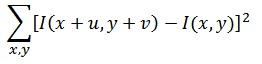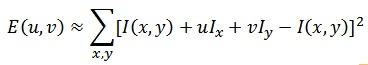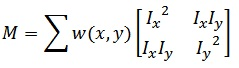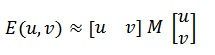## Fundamentals of Features and Corners

A lot of computer vision algorithms use features as their backbone. But what exactly is a feature?

## Harris Corner Detector

Series: Fundamentals of Features and Corners:

The Harris Corner Detector is a mathematical operator that finds features (what are features?) in an image. It is simple to compute, and is fast enough to work on computers. Also, it is popular because it is rotation, scale and illumination variation independent. However, the Shi-Tomasi corner detector, the one implemented in OpenCV, is an improvement of this corner detector.

## The mathematics

To define the Harris corner detector, we have to go into a bit of math. We'll get into a bit of calculus, some matrix math, but trust me, it won't be tough. I'll make everything easy to understand!

Our aim is to find little patches of image (or "windows") that generate a large variation when moved around. Have a look at this image:

The red square is the window we've chosen. Moving it around doesn't show much of variation. That is, the difference between the window, and the original image below it is very low. So you can't really tell if the window "belongs" to that position.

Of course, if you move the window too much, like onto the reddish region, you're bound to see a big difference. But we've moved the window too much. Not good.

Now have a look at this:

See? Even the little movement of the window produces a noticeable difference. This is the kind of window we're looking for. Here's how it translates mathematically:

• E is the difference between the original and the moved window.
• u is the window's displacement in the x direction
• v is the window's displacement in the y direction
• w(x, y) is the window at position (x, y). This acts like a mask. Ensuring that only the desired window is used.
• I is the intensity of the image at a position (x, y)
• I(x+u, y+v) is the intensity of the moved window
• I(x, y) is the intensity of the original

We've looking for windows that produce a large E value. To do that, we need to high values of the terms inside the square brackets.

(Note: There's a little error in these equations. Can you figure it out? Answer below!)

So, we maximize this term:Then, we expand this term using the Taylor series. Whats that? It's just a way of rewriting this term in using its derivatives.See how the I(x+u, y+v) changed into a totally different form ( I(x,y)+uIx + vIy )? Thats the Taylor series in action. And because the Taylor series is infinite, we've ignored all terms after the first three. It gives a pretty good approximation. But it isn't the actual value.

Next, we expand the square. The I(x,y) cancels out, so its just two terms we need to square. It looks like this:Now this messy equation can be tucked up into a neat little matrix form like this:See how the entire equation gets converted into a neat little matrix!

(The error: There's no w(x, y) in these errors :P )

Now, we rename the summed-matrix, and put it to be M:So the equation now becomes:Looks so neat after all the clutter we did above.

## Interesting windows

It was figured out that eigenvalues of the matrix can help determine the suitability of a window. A score, R, is calculated for each window:All windows that have a score R greater than a certain value are corners. They are good tracking points.

## Summary

The Harris Corner Detector is just a mathematical way of determining which windows produce large variations when moved in any direction. With each window, a score R is associated. Based on this score, you can figure out which ones are corners and which ones are not.

OpenCV implements an improved version of this corner detector. It is called the Shi-Tomasi corner detector.

## More in the series

This tutorial is part of a series called Fundamentals of Features and Corners:

#### Related posts

Utkarsh Sinha created AI Shack in 2010 and has since been working on computer vision and related fields. He is currently at Microsoft working on computer vision.